Three Phase Shield Wire Schemes Unbalance and Voltage Fluctuation Reduction Using H-Bridge Cascaded Static Synchronous Compensator (STATCOM) | OMICS International
Journal of Electrical & Electronic Systems

# Three Phase Shield Wire Schemes Unbalance and Voltage Fluctuation Reduction Using H-Bridge Cascaded Static Synchronous Compensator (STATCOM)

Alidou Koutou* and Mamadou Lamine Doumbia

Department of Electrical Engineering, Université du Québec à Trois-Rivières, Trois-Rivières, Québec, G9A 5H7, Canada

*Corresponding Author:
Alidou Koutou
Department of Electrical Engineering
Universite du Quebec a Trois-Rivieres
Tel: 15144737968
E-mail: [email protected]

Received date July 11, 2016; Accepted date July 18, 2016; Published date July 30, 2016

Citation: Koutou A, Doumbia ML (2016) Three Phase Shield Wire Schemes Unbalance and Voltage Fluctuation Reduction Using H-Bridge Cascaded Static Synchronous Compensator (STATCOM). J Electr Electron Syst 5:187. doi:10.4172/2332-0796.1000187

Copyright: © 2016 Koutou A, et al. This is an open-access article distributed under the terms of the Creative Commons Attribution License, which permits unrestricted use, distribution, and reproduction in any medium, provided the original author and source are credited.

Visit for more related articles at Journal of Electrical & Electronic Systems

#### Abstract

Unbalance and voltage fluctuation are considered as a major problem within the 3-phase Shield Wire Schemes networks (SWS). This paper deals with the methods to reduce the unbalance and voltage fluctuation by using the H-bridge cascaded Static Synchronous Compensator (STATCOM). In unconventional networks without STATCOM, the unbalance factor is generally more than 27%. In this study, we proved that after voltage compensation by the H-bridge cascaded STATCOM, unbalance factor is reduce to 0.15% for ground impedance variation. The ground resistance has been varied between 33 and 8000 Ω, and the ground inductance between 10 and 110 H. Moreover, the voltage fluctuation is reduced. The obtained results correspond to those recommended in power distribution for the proper operation of electrical equipment.

#### Keywords

Cable shielding; Power cable insulation; Distribution feeders; Earth return path, Shield Wire scheme; Voltage unbalance; Voltage fluctuation, H-bridge cascaded STATCOM

#### Introduction

The Shield Wire Scheme (SWS) is a technical method to supply power to the villages and communities located along the High Voltage (HV) lines, up to 100 km distant from the HV transforming stations.

The SWS consists of insulating the shield wires (SWs) from the towers of the HV lines and energizing the SWs with Medium Voltage (MV) (20-34.5 kV) from the HV/MV transformer station at one end of the HV line  (Figure 1). This technique reduces the cost of investments while adapting to the low power requirements of these communities . Supply of the villages along the HV line through fused single phase or three-phase transformers, depends on the scheme chosen and on the nature of the load to be supplied . The overruling criterion has been to propose solutions that require only conventional distribution equipment, without power electronic devices, to provide a reliable service with simple and ordinary operational methods.

In fact, the three-Phase SWS is an unsymmetrical system with voltage fluctuation; because the three-Phase SWS supplying conventional MV/LV distribution transformers operated with one MV terminal permanently grounded . Moreover, it has been reported that the drying up of land leads to an uncontrolled increase in grounding impedance . However, the limit values for this increase are still unknown. Increasing the value of the impedance results in a high voltage unbalance which engenders a degradation of the unbalance factor of up to 8.18% for resistance variation and 27.31% for the inductance variation . The value of unbalance factor must be contained within 1-2% for a correct operation of equipment .

The balancing of voltage can be obtained by applying different methods. But all, use only passive components, using a series resistorreactor in the earth path and capacitors connected between the two SWs and between each SW and ground . While these techniques allow the reduction of voltage unbalance in three-phase Shield wire, these types of passive compensators do not fit when a voltage variation occurs. They are functional only for predefined unbalance conditions.

To address this problem, this paper presents the use of H-bridge STATCOM to reduce unbalance and voltage fluctuation on the threephase shield wire.

Matlab/Simulink software has been used to simulate the threephase Shield wire of 34.5 KV and, a significant improvement of the unbalance and voltage fluctuation rate is obtained.

Investigated three-phase shield wire system

Three phase shield wire scheme description: Figure 1 presents a general representation of three-phase Shield Wire Scheme. A 34.5 kV shield wires medium voltage is used to provide 145/240 V. A mediumvoltage transformer terminals MV/LV cables are connected to two cables and the third cable is grounded by an electrode that contains a resistance and an inductance (Figure 2).

The three-Phase SWS is planned to be implemented in Western Africa. It has a HV 225kV-50Hz-338 km long transmission line with an intermediate substation at 134 km from the sending end. The 225kV line is equipped with AAAC conductors with Φ=31.04 mm, R20°C=0.0583 Ω/km, whereas the SWs are ACSR conductors with diameter=10.02 mm, R20°C=0.58 Ω/km .

Cascade H-Bridge Topology and Control: STATCOM H-bridge can use in the SWS network to increase the system performance (unbalance and voltage fluctuation). The interest for multilevel CHB converters comes from their capacity to provide higher power, to generate good quality of waveforms, to operate at low switching frequency with low loss of energy and the low effort on statics devices . The cascade H-bridge (CHB) STATCOM topology considers the series connection of several H-Bridges modules in order to share the total load voltage among all the modules. This allows a low voltage rating of the semiconductor devices (Figure 3).

The Sinusoidal PWM (SPWM) control method is used for this application. It is one of the most widely used control method in VSCs owing to its simplified mathematical requirements and easy implementation even with basic microcontrollers. The SPWM method is based on comparing a sinusoidal modulating signal with a triangular carrier waveform that is arranged according to required switching outputs [11,12] (Figures 4 and 5).

#### Methodology

A mathematical analysis and simulation were used. In mathematical analysis the technical characteristics of the network and the existing theories have served to determine the essential parameters for the functioning of the integrated network STATCOM. Elements of Matlab Simulink library Simpower Systems were used for the simulation. For the simulation we have given different values for the impedance and raise the voltage on the SWSs line. The unbalance factor was calculated from the values of voltage composed by the method proposed in the literature .

Passive filter’s design

As any other voltage controlled source (VCS) based topology, this configuration needs a passive filter in order to absorb the instantaneous voltage differences between the converter and the grid, and to reduce the harmonic content of the injected AC current.

Applying the voltage law in the AC side for the phase a of the STATCOM, the passive filter equation is obtained .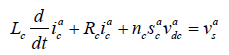(1)

Where,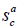is the commutation function of modules of phase a and the resistor Rc is the parasitic (series) resistance of the inductor Lc.

Input voltage calculation

In order to evaluate the stationary behavior of the CHB STATCOM, the state variable equations must be evaluated in steady state .

#### Results and analysis of simulation

The Figure 5 has been implemented in Matlab/Simulink/ SimPowerSystems. The nominal voltage Vn at the bus bar is 34.5 kV. The impact of variation of grounded resistor and inductance are evaluated .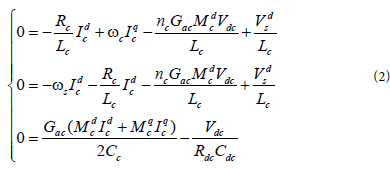Where the variables in capital letters denote the constant value of each variable in an arbitrary operating point. The voltage should ensure that the summation of the DC voltages of all the power cells must be higher than the amplitude |Vs| of the source voltage. This constraint rises because VCSs operate with a DC voltage Vdc that must be higher than the peak value of the voltage in the AC side. A suitable value for Vdc should consider at least a 10% security margin. Then Vdc is: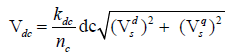(3)

Where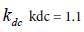to ensure the 10% margin.

Case study 1: Variation of resistor

The grounded resistance is varied from 33 to 8 000 Ω (Figure 6). The system’s three phase-to-phase voltages with passive components (capacitor bank). The rated voltage Vn is 34.5 kV. Grounded resistance variation affects the phase-to-phase voltage Vab, Vbc and Vca. The voltage rise or reduction varies +1.3% (for Vab) to -6.6% (for Vbc). The most voltage reduction on Vbc and Vca is due to the connection of phase C to the ground. The voltage unbalance factor varies from 2.8% to 8.18% (Figure 7).

In this case, the voltage amplitude variations varies between +0.37% (for Vab) and -7.33% (for Vca) (Figure 8). The use of the cascade H-bridge STATCOM makes an improvement in unbalance factor which varies between 0.15% and 3.24% (Figure 9).

Figure 8:Voltage amplitude with cascaded H-bridge STATCOM: The system’s three phase-to-phase voltages with cascaded H-bridge STATCOM.

Figure 12:Voltage amplitude with H-bridge STATCOM cascaded: the system’s three phase-to-phase voltages with cascaded H-bridge STATCOM.

Case study 2: Variation of the inductance

The grounded inductance is varied from 10 to 110 H (Figure 10). The rated voltage Vn is 34.5 kV. Grounded inductance variation affects the phase-to-phase voltage Vab, Vbc and Vca. The voltage rise or reduction varies +1.3% (for Vab) to -23.3% (for Vca). The most voltage reduction on Vca and Vbc is due to the connection of phase C to the ground. The voltage unbalance factor varies from 3.56% to 27.31% which is well above standard values (Figure 11).

In this case, the voltage amplitude variations varies between -5.31% (for Vca) and +31.59% (for Vbc) (Figure 12). The use of the cascade H-bridge STATCOM makes an improvement in unbalance factor which varies between 0.2% and 2.31% (Figure 13).

#### Conclusion

In this paper, we investigated the impact of the use of H-bridge cascaded STATCOM to reduce unbalance and voltage fluctuation on the three-phase shield wire. The unbalance and voltage fluctuation are created by the impedance difference.

The results show that the use of a STATCOM improves significantly the quality of the voltage of three-phase shield, with a very similar unbalance factor at acceptable limit compared to case when the passive components (capacitor bank) are used.

The variation of the inductance has a greater effect on the unbalance as that of the resistor. The method of unbalance reduction by H-bridge cascaded STATCOM is more efficient than passive components. From this study, we can conclude that the use of STATCOM can provide technical means to limit excessive voltage variation in SWS systems to ensure service’s quality.

#### References

Select your language of interest to view the total content in your interested language

### Article Usage

• Total views: 10982
• [From(publication date):
September-2016 - Jan 28, 2020]
• Breakdown by view type
• HTML page views : 10787Can't read the image? click here to refresh